# Decimal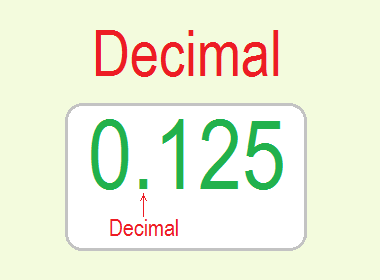Fractions having denominators 10, 100, 1000, etc. are called decimal fractions or Decimals. For example, 9/10, 68/100, and 565/1000 are decimal fractions but they are written as 0.9, 0.68, and 0.565 respectively using a dot (.) called a decimal point.

Consider the number 65423.9871. We can put this number in a place value chart. The place value chart consists of two parts as whole number part and the decimal number part. The place value chart is given here below.

## Place Value Chart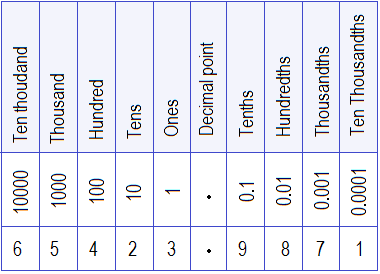To separate whole numbers from fractional numbers a small dot (.) called a decimal point is put between the ones and tenths place as shown in the number 65423.9871 above.

## Fraction and Decimal

There is a close relation between fractions and decimals. Fractions and decimals can be interchanged with each other. For example, an Easy way to change fractions into decimals is given in the table below: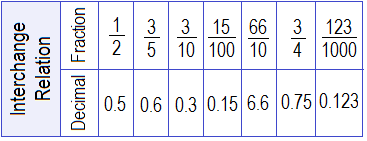So, let’s make the denominator 10, 100, 1000, 10000, and so on by multiplying the numerator and denominator by the same number. Then count the number of zeros in the denominator to count the place to keep the decimal in the numerator in the direction right to left.

********************

********************

## Workout Examples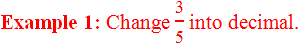Solution:

Here,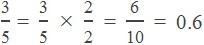Example 2: Change 0.123 into a fraction.

Solution:

Here, in 0.123, we have 3 digits after the decimal. So, we put 1 and three zeros in the denominator then remove decimal point 123 in the numerator.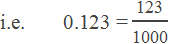You can ask your questions or problems regarding decimals and fractions in the comment section below.